International
Tables for
Crystallography
Volume D
Physical properties of crystals
Edited by A. Authier

International Tables for Crystallography (2006). Vol. D, ch. 2.2, p. 297

## Section 2.2.5. The free-electron (Sommerfeld) model

K. Schwarza*

aInstitut für Materialchemie, Technische Universität Wien, Getreidemarkt 9/165-TC, A-1060 Vienna, Austria
Correspondence e-mail: kschwarz@theochem.tuwein.ac.at

### 2.2.5. The free-electron (Sommerfeld) model

| top | pdf |

The free-electron model corresponds to the special case of taking a constant potential in the Schrödinger equation (2.2.4.1). The physical picture relies on the assumption that the (metallic) valence electrons can move freely in the field of the positively charged nuclei and the tightly bound core electrons. Each valence electron moves in a potential which is nearly constant due to the screening of the remaining valence electrons. This situation can be idealized by assuming the potential to be constant [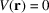]. This simple picture represents a crude model for simple metals but has its importance mainly because the corresponding equation can be solved analytically. By rewriting equation (2.2.4.1), we have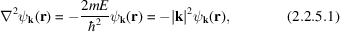where in the last step the constants are abbreviated (for later convenience) by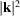. The solutions of this equation are plane waves (PWs)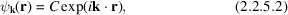where C is a normalization constant which is defined from the integral over one unit cell with volume. The PWs satisfy the Bloch condition and can be written (using the bra–ket notation) as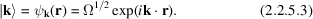From (2.2.5.1)we see that the corresponding energy (labelled by) is given by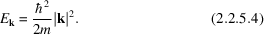In this context it is useful to consider the momentum of the electron, which classically is the vector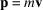, where m andare the mass and velocity, respectively. In quantum mechanics we must replaceby the corresponding operator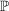.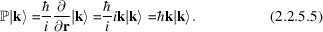Thus a PW is an eigenfunction of the momentum operator with eigenvalue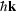. Therefore thevector is also called the momentum vector. Note that this is strictly true for a vanishing potential but is otherwise only approximately true (referred to as pseudomomentum).

Another feature of a PW is that its phase is constant in a plane perpendicular to the vector(see Fig. 2.2.5.1). For this purpose, consider a periodic function in space and time,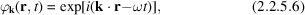which has a constant phase factor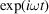within such a plane. We can characterize the spatial part bywithin this plane. Taking the nearest parallel plane (with vector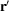) for which the same phase factors occur again but at a distanceaway (with the unit vectornormal to the plane),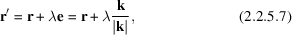then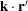must differ from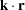by. This is easily obtained from (2.2.5.7)by multiplication withleading to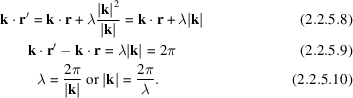Consequentlyis the wavelength and thus thevector is called the wavevector or propagation vector.Figure 2.2.5.1 | top | pdf |Plane waves. The wavevector k and the unit vector e are normal to the two planes and the vectors r in plane 1 andin plane 2.